HUIJZER.XYZ

# Nested cross-validation

2021-10-27

Nested cross-validation is said to be an improvement over cross-validation. Unfortunately, I found most explanations quite confusing, so decided to simulate some data and see what happens.

In this post, I simulate two models: one linear model which perfectly fits the data and one which overfits the data. Next, cross-validation and nested cross-validation are plotted. To keep the post short, I've hidden the code to produce the plots.

begin
import MLJLinearModels
import MLJDecisionTreeInterface

using DataFrames: DataFrame, select, Not
using Distributions: Normal
using CairoMakie: Axis, Figure, lines, lines!, scatter, scatter!, current_figure, axislegend, help, linkxaxes!, linkyaxes!, xlabel!, density, density!, hidedecorations!, violin!, boxplot!, hidexdecorations!, hideydecorations!
using MLJ: CV, evaluate, models, matching, @load, machine, fit!, predict, predict_mode, rms
using Random: seed!
using Statistics: mean, std, var, median
using MLJTuning: TunedModel, Explicit
using MLJModelInterface: Probabilistic, Deterministic
end
y_true(x) = 2x + 10;
y_real(x) = y_true(x) + rand(Normal(0, 40));
indexes = 1.0:100;
df = let
seed!(0)
DataFrame(x = indexes, y = y_real.(indexes))
end
xy
11.049.7188
22.019.3569
33.077.0028
44.022.956
55.0-28.2309
66.034.4727
77.014.6143
88.0-17.4941
99.046.4924
1010.026.7763
...
100100.0174.396
LinearModel = @load LinearRegressor pkg=MLJLinearModels verbosity=0;
TreeModel = @load DecisionTreeRegressor pkg=DecisionTree verbosity=0;
X, y = (select(df, Not(:y)), df.y);
function linear_model()
model = LinearModel(fit_intercept=true)
mach = machine(model, X, y)
fit!(mach)
return mach
end;
function tree_model()
model = TreeModel()
mach = machine(model, X, y)
fit!(mach)
return mach
end;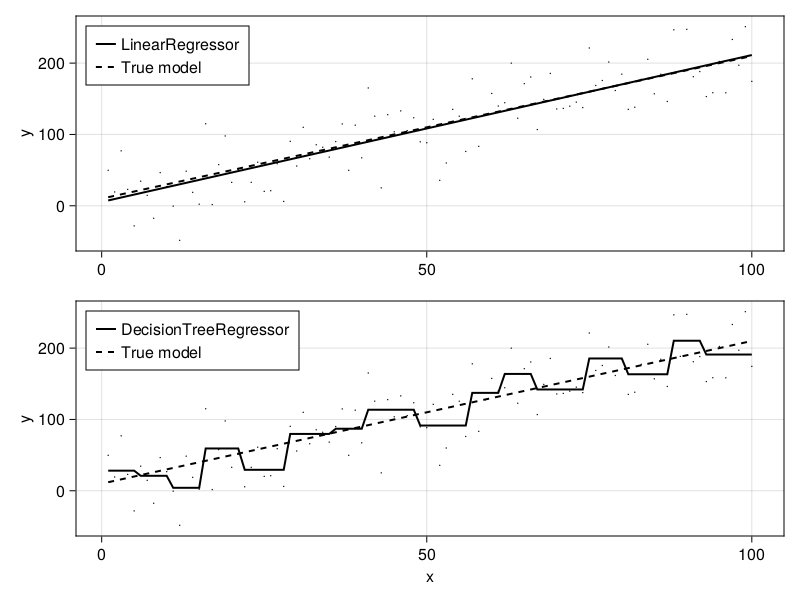Okay, so which model performs better. I would guess the LinearRegressor, but let's see what the root-mean-square error (RMS) is when we fit the models on the training data:

rms(predict(linear_model()), df.y)
33.890044054911286
rms(predict(tree_model()), df.y)
28.803941812874005

Clearly, the tree model is overfitting the data. In other words, the model is not expected to perform well on new data.

Now the question is whether we can determine that the linear model is the right one via cross-validation. Let's first plot the error for each of our $k$ folds.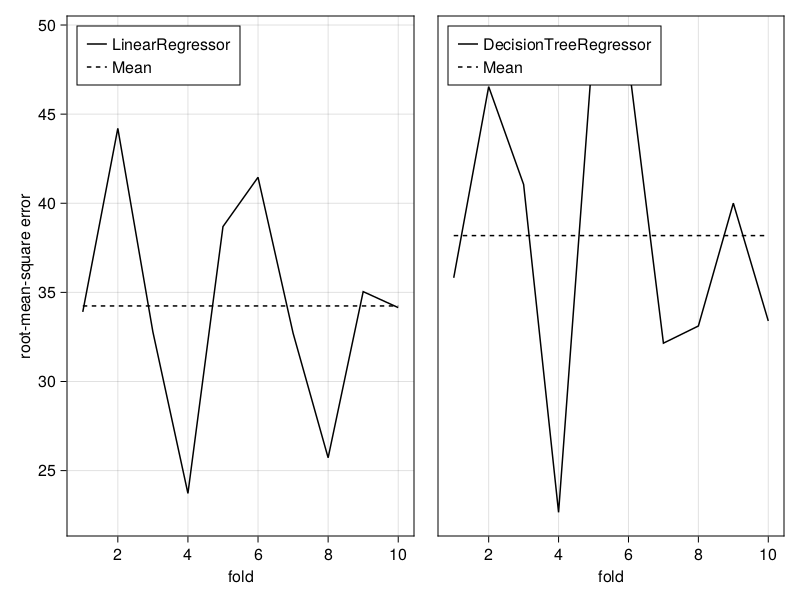So, basically cross-validation isn't gonna be perfect. If the data or standard deviation would have been different, then another model could have obtained a lower error according to the cross-validation.

Let's tryout nested cross-validation.

According to Zhang (2015), repeated 50- and 20- fold CV is best for $n_t$ sample points and the best cross-validation parameters for model selection are not necessarily the same the best cross-validation parameters for performance estimation (p. 104 and p. 105).

function evaluate_inner_folds(nfolds::Int, ntrials::Int)
inner_resampling = CV(; nfolds=nfolds)
multi_model = TunedModel(; models=[LinearModel(), TreeModel()], resampling=inner_resampling);
outer_resampling = CV(; nfolds=ntrials)
e = evaluate(multi_model, X, y; measure=rms, resampling=outer_resampling)
return e
end;

The problem of cross-validation is that it is still possible to overfit during model selection. Therefore, the only reliable way to estimate model performance is to use nested cross-validation (Krstajic et al., 2014). Also, repeated k-fold nested cross-validation is the most promising for prediction error estimation.

Let's see how the plots for nested cross-validation look:

e = evaluate_inner_folds(20, 20);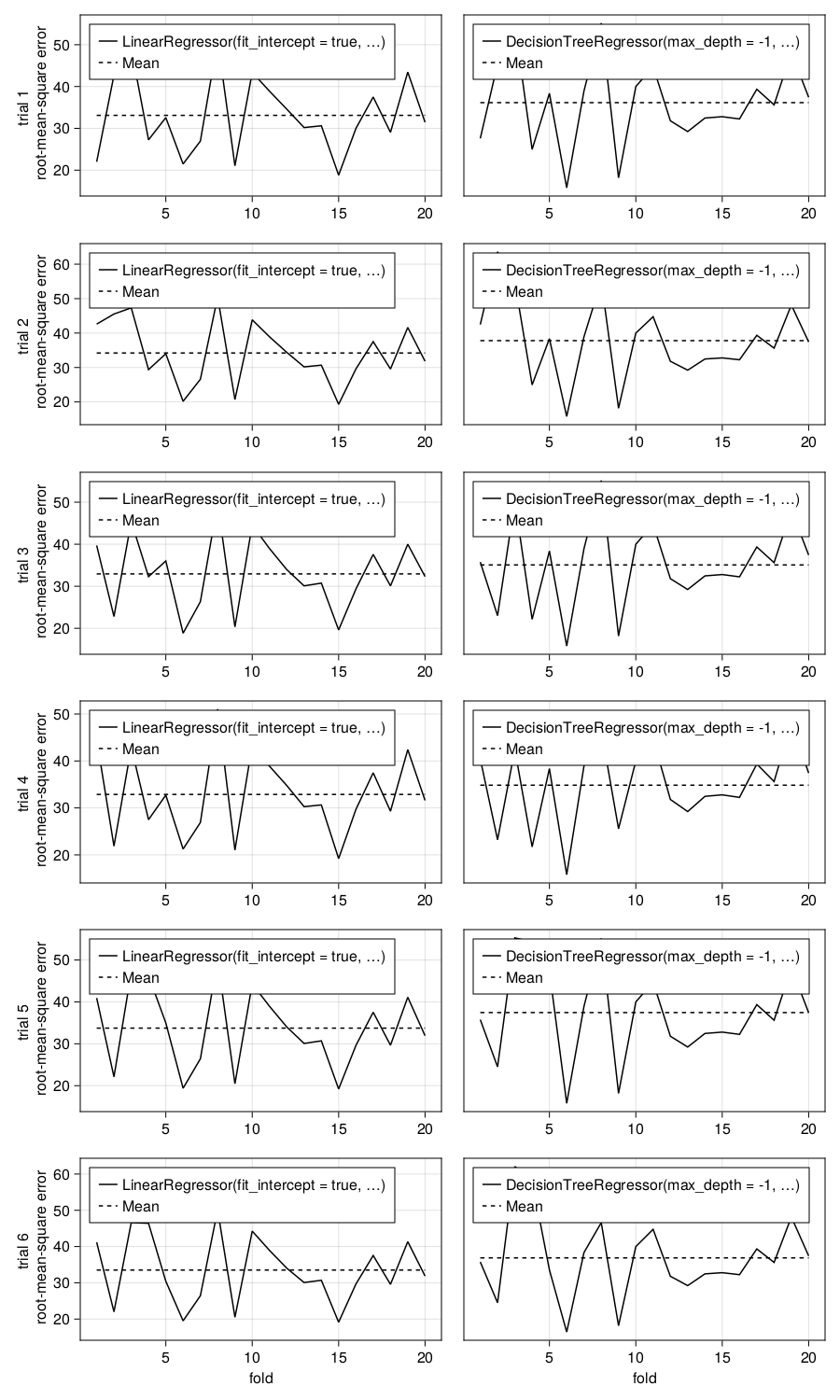That looks interesting, but what happens with the median, mean and variance if we change the number of folds and trials?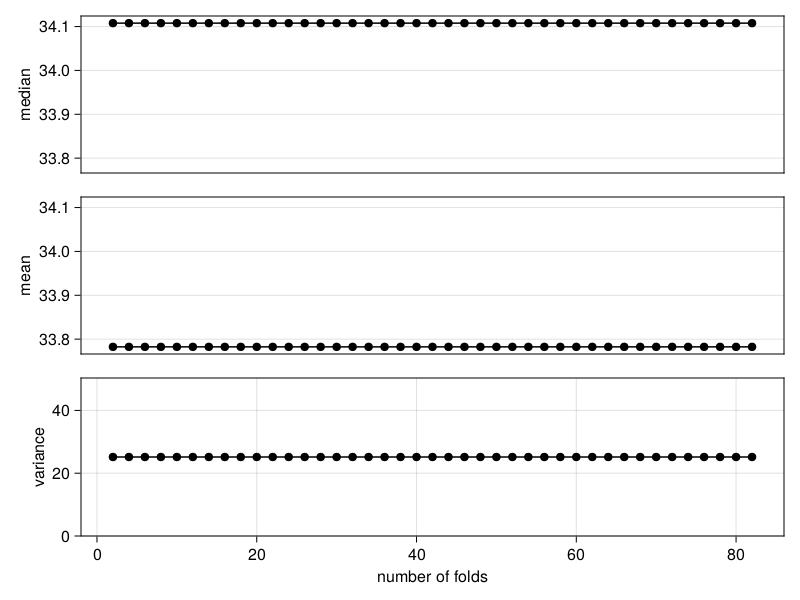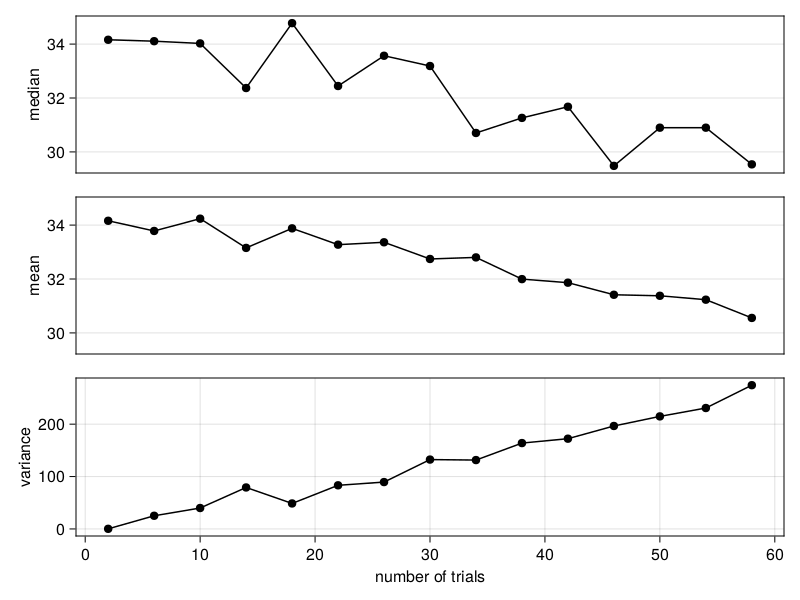I don't know what should be the take-away here. What kind of makes sense is that the variance increases for many trails. The reason is most likely that the samples become too small and fitting a model is either a complete hit or miss.

Why the median and mean go down is unclear to me. Maybe, fitting is more likely to be a hit than a miss. Therefore, if the number of trails is increased, then more fits are a hit which result in a lower error on average.

Built with Julia 1.9.0 and

CairoMakie 0.10.5
DataFrames 1.5.0
Distributions 0.25.93
MLJ 0.19.1
MLJDecisionTreeInterface 0.4.0
MLJLinearModels 0.9.1
MLJModelInterface 1.8.0
MLJTuning 0.7.4
Statistics 1.9.0

To run this blog post locally, open this notebook with Pluto.jl.